# Hexagon

A hexagon is a polygon with 6 sides and 6 angles, (hexa- means six). In the figure below are 3 different types of hexagons.

A hexagon is a shape that is commonly seen in everyday life. The shapes that make up a honeycomb, a nut, and bolts are all examples of real life objects in the shape of hexagon.

## Hexagon classifications

Like other polygons, a hexagon can be classified as regular or irregular. If all the sides and interior angles of a hexagon are equal, it is a regular hexagon. Otherwise it is an irregular hexagon.

Regular hexagonIrregular hexagon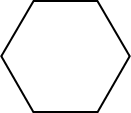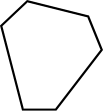All sides and interior angles are equal Not all sides and angles are equal

Hexagons or other polygons can also be classified as either convex or concave. If all interior angles of a hexagon or polygon are less than 180°, it is convex. If one or more interior angles are larger than 180°, it is concave. A regular hexagon is always a convex hexagon.

Convex hexagonConcave hexagon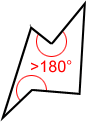All interior angles < 180° One or more interior angles > 180°

## Diagonals of hexagon

A diagonal is a line segment joining two non-consecutive vertices. Three diagonals can be drawn from each vertex. A total of nine diagonals can be drawn for a hexagon. The following figure is an example.

## Internal angles of a hexagon

The sum of the interior angles of a hexagon equals 720°.

As shown in the figure above, three diagonals can be drawn to divide the hexagon into four triangles. The blue lines above show just one way to divide the hexagon into triangles; there are others. The sum of interior angles of the four triangles equals the sum of interior angles of the hexagon. Since the sum of the interior angles of a triangle is 180°, the sum of the interior angles of the hexagon is 4 × 180° = 720°.

## Regular hexagon

A regular hexagon is a hexagon in which all sides have equal length and all interior angles have equal measure.

### Angles and sides of a regular hexagon

Since each of the six interior angles in a regular hexagon are equal in measure, each interior angle measures 720°/6 = 120°, as shown below.

Each exterior angle of a regular hexagon has an equal measure of 60°.

Sides of a regular hexagon are equal in length and opposite sides are parallel.

### Symmetry in a regular hexagon

A regular hexagon has 6 lines of symmetry and a rotational symmetry of order 6, which means that it can be rotated in such a way that it will look the same as the original shape 6 times in 360°.

Lines of symmetryRotational symmetry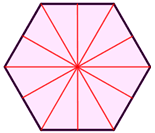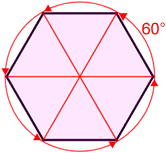6 lines of symmetry Six 60° angles of rotation

### Area of a regular hexagon

The area of a regular hexagon with side length s is:From the center, a regular hexagon can be divided into six equilateral triangles, each having side length, s, as shown below.

The area, T, of one of the equilateral triangles, drawn in blue, can be found by using, where the apothem is the height of the triangle. Since there are six equilateral triangles, the area of a regular hexagon is.

Example:

Find the area of a regular hexagon that has a side length of 8.

Using: